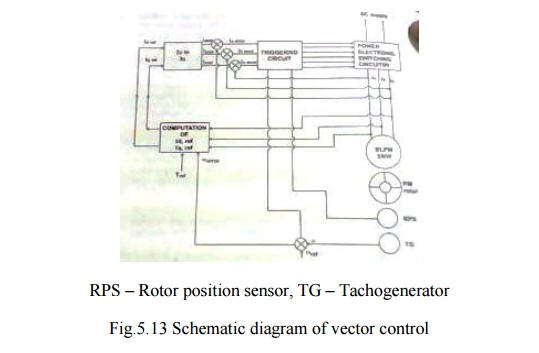Home | | Special Electrical Machines | Vector Control of BLPM SNW Motor

# Vector Control of BLPM SNW Motor

1. Principle of vector control 2. Schematic Diagram of Vector Control

VECTOR CONTROL OF BLPM SNW MOTOR

Electromagnetic torque in any electrical machine is developed due to the interaction of current carrying armature conductors with the air gap flux. Consider a two machine whose armature conductor currents and air gap flux are as shown in fig. 5.12. Here the flux is in quadrature with the armature mmf axis.Each and every armature conductor experiences a force which contributes the torque. The torque contributed by various armature conductors have the same direction even through their magnitude may vary. It is observed that the steady state and dynamic (behaviors) performance of a most of such an arrangement are better.

Consider a second case wherein the armature conductor current distribution and air gap flux distribution are as shown in fig. 6.26. In this case the angle between the axis of the air gap flux and the armature mmf axis is different from 90° elec.

In this case also each and every armature conductor experiences a force and contributes to the torque. But in this case the direction of the torque experienced by the conductors is not the same. Since conduction develops torque in one direction while the others develop in the opposite direction. As a result, the resultant torque gets reduced; consequently it is observed that both the steady state and dynamic performance of such a motor is poorer.

For a BLPM motor to have better steady state and dynamic performance, it is essential that the armature mmf axis and the axis of PM are to be in quadrature for all operating condition.

1. Principle of vector control

BLPM SNW motor is usually employed for variable speed applications. For this we keep V/f constant and vary V and f to get the desired speed and torque.

From the theory of BLPM SNW motor it is known that as the speed is varied from a very low value upto the corner frequency, the desired operating point of current is such that Id =0 and I is along the q-axis. Such a condition can be achieved by suitably controlling the voltage by PWM technique after adjusting the frequency to a desired value.

When the frequency is more than the corner frequency it is not possible to make Id =0, due to the voltage constraints. In such a case a better operating point for current is obtained with minimum Id value after satisfying the voltage constraints. Controlling BLPM SNW motor taking into consideration the above mentioned aspects is known as ―vector Control‖ of BLPM SNW motor.

2. Schematic Diagram of Vector Control

The schematic block diagram of vector control is as shown in figure 5.13Knowing the value of the desired torque and speed and also the parameters and the voltage to which the motor is subjected to, it is possible to complete the values of id .ref and iq .ref for the desired dynamic and steady state performance.The reference values of id and iq are transformed into reference values of currents namely ia ref, ib ref and ic ref. These currents are compared with the actual currents and the error values actuate the triggering circuitry which is also influenced by the rotor position sensor and speed. Thus the vector control of BLPM SNW motor is achieved.

Study Material, Lecturing Notes, Assignment, Reference, Wiki description explanation, brief detail
Special Electrical Machines : Permanent Magnet Synchronous Motor : Vector Control of BLPM SNW Motor |